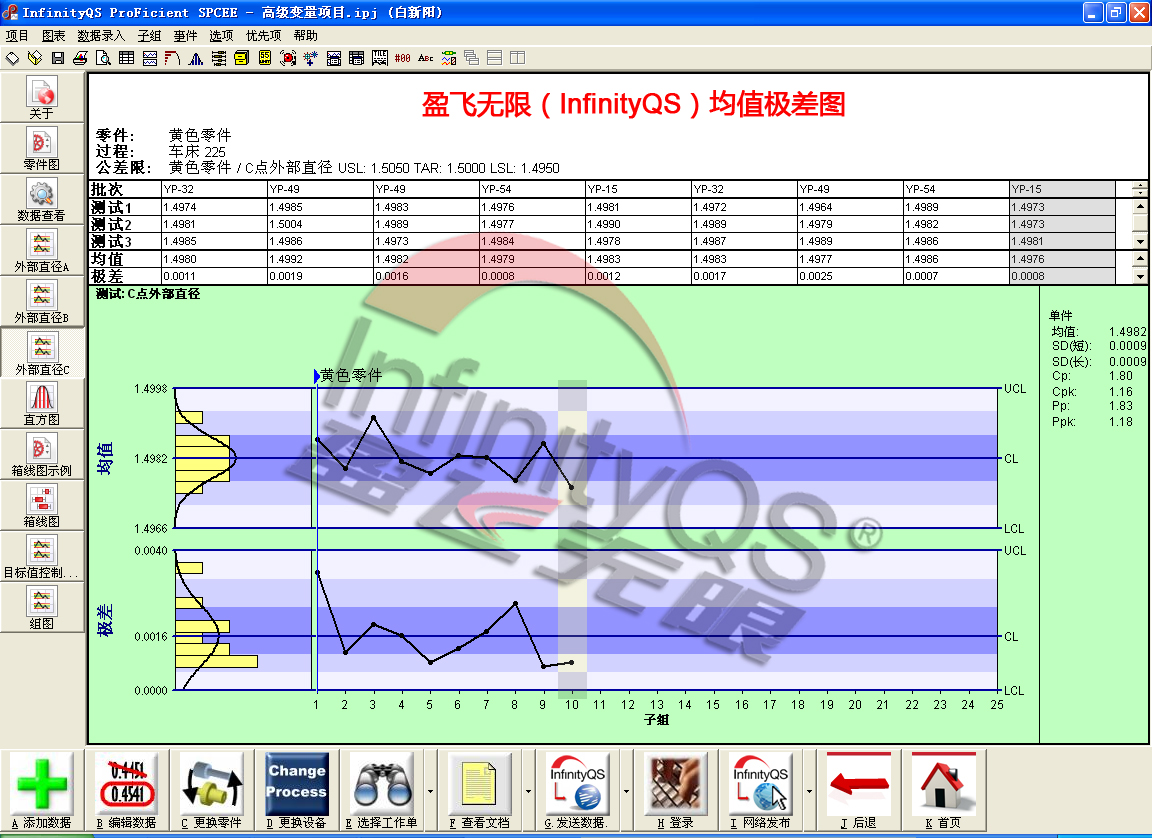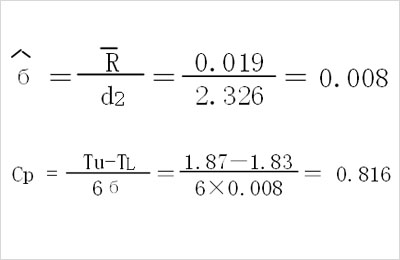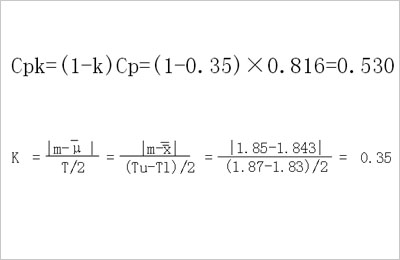# SPC控制图公式_均值极差SPC控制图公式应用实例分析

SPC控制图公式是指用来制作SPC控制图的各种公式的总和，控制图不同，公式也不同。下面我们就以一实例，讲解制作均值极差控制图都需要用到哪些SPC控制图公式。UCLR=D4R=2.114×0.019=0.040

CLR=R=0.019

LCLR=D3R=0×0.019=0 (A2、D3和D4是常数查表可得0.577、0、2.114）

UCLX=X+A2R=1.843+0.577×0.019=1.852

CLX=X=1.843

LCLX=X-A2R=1.843-0.577×0.019=1.832